# Sharpe Ratio

## Distribution of Maximal Sharpe, the Markowitz Approximation

In a previous blog post we looked at a statistical test for overfitting of trading strategies proposed by Lopez de Prado, which essentially uses a $$t$$-test threshold on the maximal Sharpe of backtested returns based on assumed independence of the returns. (Actually it is not clear if Lopez de Prado suggests a $$t$$-test or relies on approximate normality of the $$t$$, but they are close enough.) In that blog post, we found that in the presence of mutual positive correlation of the strategies, the test would be somewhat conservative. It is hard to say just how conservative the test would be without making some assumptions about the situations in which it would be used.

This is a trivial point, but needs to be mentioned: to create a useful test of strategy overfitting, one should consider how strategies are developed and overfit. There are numerous ways that trading strategies are, or could be developed. I will enumerate some here, roughly in order of decreasing methodological purity:

1. Alice the Quant goes into the desert on a Vision Quest. She emerges three days later with a fully formed trading idea, and backtests it a single time to satisfy the investment committee. The strategy is traded unconditional on the results of that backtest.

2. Bob the Quant develops a black box that generates, on demand, a quantitative trading strategy, and performs a backtest on that strategy to produce an unbiased estimate of the historical performance of the strategy. All strategies are produced de novo, without any relation to any other strategy ever developed, and all have independent returns. The black box can be queried ad infinitum. (This is essentially Lopez de Prado's assumed mode of development.)

3. The same as above, but the strategies possibly have correlated returns, or were possibly seeded by published anomalies or trading ideas.

4. Carole the Quant produces a single new trading idea, in a white box, that is parametrized by a number of free parameters. The strategy is backtested on many settings of those parameters, which are chosen by some kind of design, and the settings which produce the maximal Sharpe are selected.

5. The same as above, except the parameters are optimized based on backtested Sharpe using some kind of hill-climbing heuristic or an optimizer.

6. The same as above, except the trading strategy was generally known and possibly overfit by other parties prior to publication as "an anomaly".

7. Doug the Quant develops a gray box trading idea, adding and removing parameters while backtesting the strategy and debugging the code, mixing machine and human heuristics, and leaving no record of the entire process.

8. A small group of Quants separately develop a bunch of trading strategies, using common data and tools, but otherwise independently hillclimb the in-sample Sharpe, adding and removing parameters, each backtesting countless unknown numbers of times, all in competition to have money allocated to their strategies.

9. The same, except the fund needs to have a 'good quarter', otherwise investors will pull their money, and they really mean it this time.

The first development mode is intentionally ludicrous. (In fact, these modes are also roughly ordered by increasing realism.) It is the only development model that might result in underfitting. The division between the second and third modes is loosely quantifiable by the mutual correlation among strategies, as considered in the previous blog post. But it is not at all clear how to approach the remaining development modes with the maximal Sharpe statistic. Perhaps a "number of pseudo-independent backtests" could be estimated and then used with the proposed test, but one cannot say how this would work with in-sample optimization, or the diversification benefit of looking in multidimensional parameter space.

## The Markowitz Approximation

Perhaps the maximal Sharpe test can be salvaged, but I come to bury Caesar, not to resuscitate him. Some years ago, I developed a test for overfitting based on an approximate portfolio problem. I am ashamed to say, however, that while writing this blog post I have discovered that this approximation is not as accurate as I had remembered! It is interesting enough to present, I think, warts and all.

Suppose you could observe the time series of backtested returns from all the backtests considered. By 'all', I want to be very inclusive if the parameters were somehow optimized by some closed form equation, say. Let $$Y$$ be the $$n \times k$$ matrix of returns, with each row a date, and each column one of the backtests. We suppose we have selected the strategy which maximizes Sharpe, which corresponds to picking the column of $$Y$$ with the largest Sharpe.

Now perform some kind of dimensionality reduction on the matrix $$Y$$ to arrive at

$$Y \approx X W,$$

where $$X$$ is an $$n \times l$$ matrix, and $$W$$ is an $$l \times k$$ matrix, and where $$l \ll k$$. The columns of $$X$$ approximately span the columns of $$Y$$. Picking the strategy with maximal Sharpe now approximately corresponds to picking a column of $$W$$ that has the highest Sharpe when multiplied by $$X$$. That is, our original overfitting approximately corresponded to the optimization problem

$$\max_{w \in W} \operatorname{Sharpe}\left(X w\right).$$

The unconstrained version of this optimization problem is solved by the Markowitz portfolio. Moreover, if the returns $$X$$ are multivariate normal with independent rows, then the distribution of the (squared) Sharpe of the Markowitz portfolio is known, both under the null hypothesis (columns of $$X$$ are all zero mean), and the alternative (the maximal achievable population Sharpe is non-zero), via Hotelling's $$T^2$$ statistic.

If $$\hat{\zeta}$$ is the (in-sample) Sharpe of the (in-sample) Markowitz portfolio on $$X$$, assumed i.i.d. Normal, then

$$\frac{(n-l) \hat{\zeta}^2}{l (n - 1)}$$

follows an F distribution with $$l$$ and $$n-l$$ degrees of freedom. I wrote the psropt and qsropt functions in SharpeR to compute the CDF and quantile of the maximal in-sample Sharpe to support this kind of analysis.

I should note there are a few problems with this approximation:

1. There is no strong theoretical basis for this approximation: we do not have a model for how correlated returns should arise for a particular population, nor what the dimension $$l$$ should be, nor what to expect under the alternative, when the true optimal strategy has positive Sharpe. (I suspect that posing overfit of backtests as a Gaussian Process might be fruitful.)

2. We have to estimate the dimensionality, $$l$$, which is about as odious as estimating the number of 'pseudo-observations' in the maximal Sharpe test. I had originally suspected that $$l$$ would be 'obvious' from the application, but this is not apparently so.

3. Although the returns may live nearly in an $$l$$ dimensional subspace, we might have have selected a suboptimal combination of them in our overfitting process. This would be of no consequence if the $$l$$ were accurately estimated, but it will stymie our testing of the approximation.

Despite these problems, let us press on.

### An example: a two window Moving Average Crossover

While writing this blog post, I went looking for examples of 'classical' technical strategies which would be ripe for overfitting (and which I could easily simulate under the null hypothesis). I was surprised to find that freely available material on Technical Analysis was even worse than I could imagine. owhere among the annotated plots with silly drawings could I find a concrete description of a trading strategy, possibly with free parameters to be fit to the data. Rather than wade through that swamp any longer, I went with an old classic, the Moving Average Crossover.

The idea is simple: compute two moving averages of the price series with different windows. When one is greater than the other, hold the asset long, otherwise hold it short. The choice of two windows must be overfit by the quant. Here I perform that experiment, but under the null hypothesis, with zero mean simulated returns generated independently of each other. Any realization of this strategy, with any choice of the windows, will have zero mean returns and thus zero Sharpe.

First I collect 'backtests' (sans any trading costs) of two window MAC for a single realization of returns where the two windows were allowed to vary from 2 to around 1000. The backtest period is 5 years of daily data. I compute the singular value decomposition of the returns, then present a scree plot of the singular values.

suppressMessages({
library(dplyr)
library(fromo)
library(svdvis)
})
# return time series of *all* backtests
backtests <- function(windows,rel_rets) {
nwin <- length(windows)
nc <- choose(nwin,2)

# log returns
log_rets <- log(1 + rel_rets)
# price series
psers <- exp(cumsum(log_rets))
avgs <- lapply(windows,fromo::running_mean,v=psers)

X <- matrix(0,nrow=length(rel_rets),ncol=2*nc)

idx <- 1
for (iii in 1:(nwin-1)) {
for (jjj in (iii+1):nwin) {
position <- sign(avgs[[iii]] - avgs[[jjj]])
myrets <- position * fwd_rets
X[,idx] <- myrets
X[,idx+1] <- -myrets
idx <- idx + 1
}
}
# trim the last row, which has the last NA
X <- X[-nrow(X),]
X
}
geomseq <- function(from=1,to=1,by=(to/from)^(1/(length.out-1)),length.out=NULL) {
if (missing(length.out)) {
lseq <- seq(log(from),log(to),by=log(by))
} else {
lseq <- seq(log(from),log(to),by=log(by),length.out=length.out)
}
exp(lseq)
}

# which windows to test
windows <- unique(ceiling(geomseq(2,1000,by=1.15)))
nobs <- ceiling(3 * 252)
maxwin <- max(windows)
rel_rets <- rnorm(maxwin + 10 + nobs,mean=0,sd=0.01)

XX <- backtests(windows,rel_rets)
# grab the last nobs rows
XX <- XX[(nrow(XX)-nobs+1):(nrow(XX)),]

# perform svd
blah <- svd(x=XX,nu=11,nv=11)
# look at it
ph <- svdvis::svd.scree(blah) +
labs(x='Singular Vectors',y='Percent Variance Explained')
print(ph)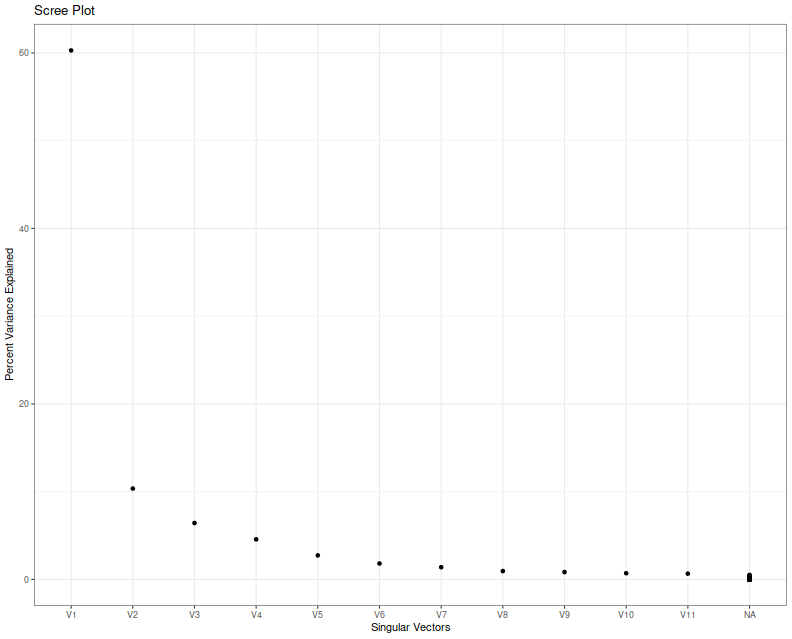I think we can agree that nobody knows how to interpret a scree plot. However, in this case a large proportion of the explained variance seems to encoded in the first two eigenvalues, which is consistent with my a priori guess that $$l=2$$ in this case because of the two free parameters.

Next I simulate overfitting, performing that same experiment, but picking the largest in-sample Sharpe ratio. I create a series of independent zero mean returns, then backtest a bunch of MAC strategies, and save the maximal Sharpe over a 3 year window of daily data. I repeat this experiment ten thousand times, and then look at the distribution of that maximal Sharpe.

suppressMessages({
library(dplyr)
library(tidyr)
library(tibble)
library(SharpeR)
library(future.apply)
library(ggplot2)
})
ope <- 252

geomseq <- function(from=1,to=1,by=(to/from)^(1/(length.out-1)),length.out=NULL) {
if (missing(length.out)) {
lseq <- seq(log(from),log(to),by=log(by))
} else {
lseq <- seq(log(from),log(to),by=log(by),length.out=length.out)
}
exp(lseq)
}
# one simulation. returns maximal Sharpe
onesim <- function(windows,n=1000) {
maxwin <- max(windows)
rel_rets <- rnorm(maxwin + 10 + n,mean=0,sd=0.01)
# log returns
log_rets <- log(1 + rel_rets)
# price series
psers <- exp(cumsum(log_rets))
avgs <- lapply(windows,fromo::running_mean,v=psers)

nwin <- length(windows)
maxsr <- 0

for (iii in 1:(nwin-1)) {
for (jjj in (iii+1):nwin) {
position <- sign(avgs[[iii]] - avgs[[jjj]])
myrets <- position * fwd_rets
# compute Sharpe on some part of this
compon <- myrets[(length(myrets)-n):(length(myrets)-1)]
thissr <- SharpeR::as.sr(compon,ope=ope)$sr # we are implicitly testing both combinations of long and short here, # so we take the absolute Sharpe, since we will always overfit to # the better of the two: maxsr <- max(maxsr,abs(thissr)) } } maxsr } windows <- unique(ceiling(geomseq(2,1000,by=1.15))) nobs <- ceiling(3 * 252) nrep <- 10000 plan(multiprocess) set.seed(1234) system.time({ simvals <- future_replicate(nrep,onesim(windows,n=nobs)) })   user system elapsed 0.722 0.189 245.765  Here I plot the empirical quantiles of the maximal (annualized) Sharpe versus theoretical quantiles under the Markowitz approximation, assuming $$l=2$$. I also plot the $$y=x$$ lines, and horizontal and vertical lines at the nominal upper $$0.05$$ cutoff based on the Markowitz approximation. # plot max value vs quantile library(ggplot2) apxdf <- 2.0 ph <- data.frame(simvals=simvals) %>% ggplot(aes(sample=simvals)) + geom_vline(xintercept=SharpeR::qsropt(0.95,df1=apxdf,df2=nobs,zeta.s=0,ope=ope),linetype=3) + geom_hline(yintercept=SharpeR::qsropt(0.95,df1=apxdf,df2=nobs,zeta.s=0,ope=ope),linetype=3) + stat_qq(distribution=SharpeR::qsropt,dparams=list(df1=apxdf,df2=nobs,zeta.s=0,ope=ope)) + geom_abline(intercept=0,slope=1,linetype=2) + labs(title='empirical quantiles of maximal Sharpe versus Markowitz Approximation', x='theoretical quantile',y='empirical quantile (Sharpe in annual units)') print(ph)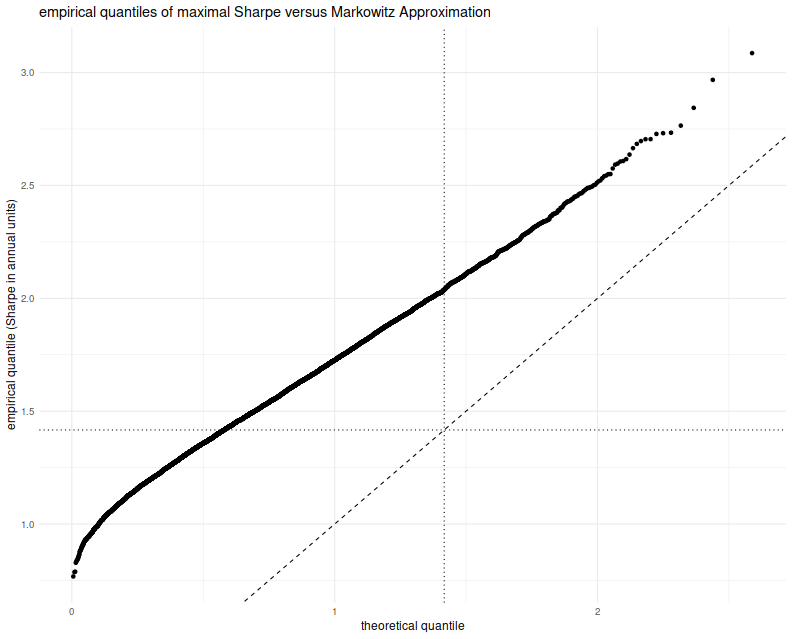This approximation is clearly no good. The empirical rate of type I errors at the $$0.05$$ level is around 60%, and the Q-Q line is just off. I must admit that when I previously looked at this approximation (and in the vignette for SharpeR!) I used the qqline function in base R, which fits a line based on the first and third quartile of the empirical fit. That corresponds to an affine shift of the line we see here, and nothing seems amiss. So perhaps the Markowitz approximation can be salvaged, if I can figure out why this shift occurs. Perhaps we have only traded picking a maximal $$t$$ for picking a maximal $$T^2$$ and there still has to be a mechanism to account for that. Or perhaps in this case, despite the 'obvious' setting of $$l=2$$, we should have chosen $$l=7$$, for which the empirical rate of type I errors is around 60%, though we have no way of seeing that 7 from the scree plot or by looking at the mechanism for generating strategies. Or perhaps the problem is that we have not actually picked a maximal strategy over the subspace, and this technique can only be used to provide a possibly conservative test. In this regard, our test would be no more useful than the maximal Sharpe test described in the previous blog post. Click to read and post comments #### Jun 14, 2018 ## Distribution of Maximal Sharpe I recently ran across what Marcos Lopez de Prado calls "The most important plot in Finance". As I am naturally antipathetic to such outsized, self-aggrandizing claims I was resistant to drawing attention to it. However, what it purports to correct is a serious problem in quantitative trading, namely backtest overfit (variously known elsewhere as data-dredging, p-hacking, etc.). Suppose you had some process that would, on demand, generate a trading strategy, backtest it, and present you (somehow) with an unbiased estimate of the historical performance of that strategy. This process might be random generation a la genetic programming, some other automated process, or a small army of very eager quants ("grad student descent"). If you had access to such a process, surely you would query it hundreds or even thousands of times, much like a slot machine, to get the best strategy. Before throwing money at the best strategy, first you have to identify it (probably via the Sharpe ratio on the backtested returns, or some other heuristic objective), then you should probably assess whether it is any good, or simply the result of "dumb luck". More formally, you might perform a hypothesis test under the null hypothesis that all the generated strategies have non-positive expected returns, or you might try to construct a confidence interval on the Signal-Noise ratio of the strategy with best in-sample Sharpe. The "Most Important Plot" in all finance is, apparently, a representation of the distribution of the maximal in-sample Sharpe ratio of $$B$$ different backtests over strategies that are zero mean, and have independent returns, versus that $$B$$. As presented by its author it is a heatmap, though I imagine boxplots or violin plots would be easier to digest. To generate this plot, note that the smallest value of $$B$$ independent uniform random variates takes a Beta distribution with parameters $$1$$ and $$B$$. So to find the $$q$$th quantile of the maximum Sharpe, compute the $$1-q$$ quantile of the $$\beta\left(1,B\right)$$ distribution, then plug that the quantile function of the Sharpe distribution with the right degrees of freedom and zero Signal Noise parameter. (See Exercise 3.29 in my Short Sharpe Course.) In theory this should give you a significance threshold against which you can compare your observed maximal Sharpe. If yours is higher, presumably you should trade the strategy (uhh, after you pay Marcos for using his patented technology), otherwise give up quantitative trading and become an accountant. One huge problem with this Most Important Plot method (besides its ignorance of entire fields of research on Multiple Hypothesis Testing, False Discovery Rate, Estimation After Selection, White's Reality Check, Romano-Wolf, Politis, Hansen's SPA, inter alia) is the assumption of independence. "Assuming quantities are independent which are not independent" is the downfall of many a statistical procedure applied in the wild (much more so than non-normality), and here is no different. And we have plenty of reason to believe returns from any real strategy generation process would fail independence: 1. Strategies are typically generated on a limited universe of assets, and using a limited set of predictive 'features', and are tested on a single, often relatively short, history. 2. Most strategy generation processes (synthetic and human) have a very limited imagination. 3. Most strategy generation processes (synthetic and human) tend to work incrementally, generating new strategies after having observed the in-sample performance of existing strategies. They "hill-climb". My initial intuition was that dependence among strategies, especially of the hill-climbing variety, would cause this Most Important Test to have a much higher rate of type I errors than advertised. (This would be bad, since it would effectively still pass dud trading strategies while selling you a false sense of security.) However, it seems that the more innocuous correlation of random generation on a limited set of strategies and assets causes this test to be conservative. (It is similar to Bonferroni's correction in this regard.) To establish this conservatism, you can use Slepian's Lemma. This lemma is a kind of stochastic dominance result for multivariate normals. It says that if $$X$$ and $$Y$$ are $$B$$-variate normal random variables, where each element is zero mean and unit variance, and if the covariance of any pair of elements of $$X$$ is no less than the covariance of the corresponding pair of elements of $$Y$$, then $$Y$$ stochastically dominates $$X$$, in the multivariate sense. This is actually a stronger result than what we need, which is stochastic dominance of the maximal element of $$Y$$ over the maximal element of $$X$$, which it implies. Here I simply illustrate this dominance empirically. I create a $$B$$-variate normal with zero mean and unit variance of marginals, for $$B=1000$$. The elements are all correlated to each other with correlation $$\rho$$. I compute the maximum over the $$B$$ elements. I perform this simulation 100 thousand times, then compute the $$q$$th empirical quantile over the $$10^5$$ maximum values. I vary $$\rho$$ from 0 to 0.75. Here is the code: suppressMessages({ library(dplyr) library(tidyr) library(tibble) library(doFuture) library(broom) library(ggplot2) }) # one (bunch of) simulation. onesim <- function(B,rho=0,propcor=1.0,nsims=100L) { propcor <- min(1.0,max(propcor,1-propcor)) rho <- abs(rho) # (anti)correlated part; each row is a simulation X0 <- outer(array(rnorm(nsims)),array(2*rbinom(B,size=1,prob=propcor)-1)) # idiosyncratic part XF <- matrix(rnorm(B*nsims),nrow=nsims,ncol=B) XX <- sqrt(rho) * X0 + sqrt(1-rho) * XF data_frame(maxval=apply(XX,1,FUN="max")) } # many sims. repsim <- function(B,rho=0,propcor=1.0,nsims=1000L) { maxper <- 100L nreps <- ceiling(nsims / maxper) jumble <- replicate(nreps,onesim(B=B,rho=rho,propcor=propcor,nsims=maxper),simplify=FALSE) %>% bind_rows() } manysim <- function(B,rho=0,propcor=1.0,nsims=10000L,nnodes=7) { if ((nsims > 100*nnodes) && require(doFuture)) { registerDoFuture() plan(multiprocess) # do in parallel. nper <- ceiling(nsims / nnodes) retv <- foreach(i=1:nnodes,.export = c('B','rho','propcor','nper','onesim','repsim')) %dopar% { repsim(B=B,rho=rho,propcor=propcor,nsims=nper) } %>% bind_rows() retv <- retv[1:nsims,] } else { retv <- repsim(B=B,rho=rho,propcor=propcor,nsims=nsims) } retv } params <- tidyr::crossing(data_frame(rho=c(0,0.25,0.5,0.75)), data_frame(propcor=c(0.5,1.0))) %>% filter(rho > 0 | propcor == 1) # run a bunch; nrep <- 1e5 set.seed(1234) system.time({ results <- params %>% group_by(rho,propcor) %>% summarize(sims=list(manysim(B=1000,rho=rho,propcor=propcor,nsims=nrep))) %>% ungroup() %>% tidyr::unnest() })   user system elapsed 142.680 3.522 27.266  # aggregate the results do_aggregate <- function(results) { results %>% group_by(rho,propcor) %>% summarize(qs=list(broom::tidy(quantile(maxval,probs=seq(0.50,0.9975,by=0.0025))))) %>% ungroup() %>% tidyr::unnest() %>% rename(qtile=names,value=x) } sumres <- results %>% do_aggregate()  Here I plot the empirical $$q$$th quantile of the maximum versus $$q$$, with different lines for the different values of $$\rho$$. I include a vertical line at the 0.95 quantile, to show where the nominal 0.05 level threshold is. The takeaway, as implied by Slepian's lemma, is that the maximum over $$B$$ Gaussian elements decreases stochastically as the correlation of elements increases. Thus when you assume independence of your backtests, you use the red line as your significance threshold (typically where it intersects the dashed vertical line), while your processes live on the green or blue or purple lines. Your test will be too conservative, and your true type I rate will be lower than the nominal rate. # plot max value vs quantile library(ggplot2) ph <- sumres %>% filter(propcor==1) %>% mutate(rho=factor(rho)) %>% mutate(xv=as.numeric(gsub('%$','',qtile))) %>%
ggplot(aes(x=xv,y=value,color=rho,group=rho)) +
geom_line() + geom_point(alpha=0.2) +
geom_vline(xintercept=95,linetype=2,alpha=0.5) +    # the 0.05 significance cutoff
labs(title='quantiles of maximum of B=1000 multivariate normal, various correlations',
x='quantile',y='maximum value')
print(ph)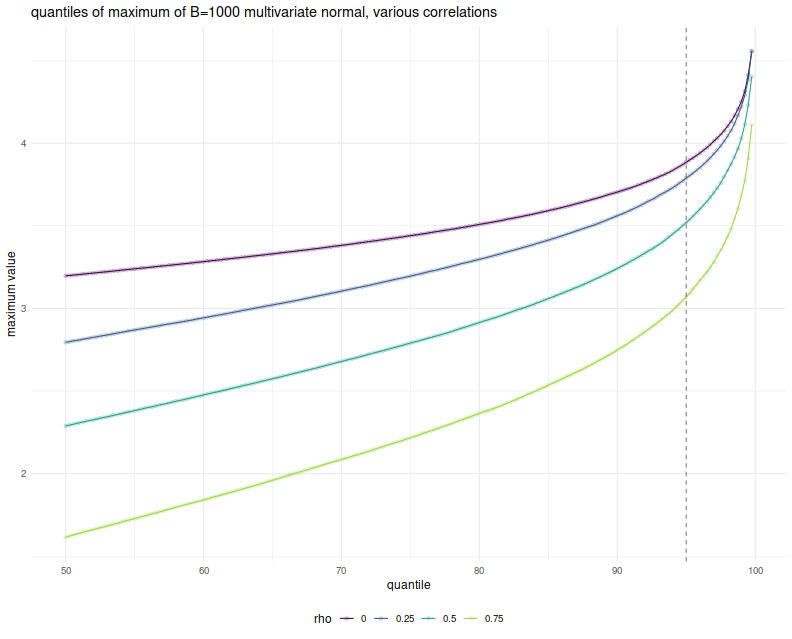But wait, these simulations were over Gaussian vectors (and Slepian's Lemma is only applicable in the Gaussian case), while the Most Important Test is to be applied to the Sharpe ratio. They have different distributions. It turns out, however, that when the population mean is the zero vector, the vector of Sharpe ratios of returns with correlation matrix $$R$$ is approximately normal with variance-covariance matrix $$\frac{1}{n} R$$. (This is in section 4.2 of Short Sharpe Course.) Here I establish that empirically, by performing the same simulations again, this time spawning 252 days of normally distributed returns with zero mean, for $$B$$ possibly correlated strategies, computing their Sharpes, and taking the maximum. I only perform $$10^4$$ simulations because this one is quite a bit slower:

# really just one simulation.
onesim <- function(B,nday,rho=0,propcor=1.0,pzeta=0) {
propcor <- min(1.0,max(propcor,1-propcor))
rho <- abs(rho)
# correlated part; each row is one day
X0 <- outer(array(rnorm(nday)),array(2*rbinom(B,size=1,prob=propcor)-1))
# idiosyncratic part
XF <- matrix(rnorm(B*nday),nrow=nday,ncol=B)
XX <- pzeta + (sqrt(rho) * X0 + sqrt(1-rho) * XF)
sr <- colMeans(XX) / apply(XX,2,FUN="sd")
# if you wanted to look at them cumulatively:
#data_frame(maxval=cummax(sr),iterate=1:B)
# otherwise just the maxval
data_frame(maxval=max(sr),iterate=B)
}
# many sims.
repsim <- function(B,nday,rho=0,propcor=1.0,pzeta=0,nsims=1000L) {
jumble <- replicate(nsims,onesim(B=B,nday=nday,rho=rho,propcor=propcor,pzeta=pzeta),simplify=FALSE) %>%
bind_rows()
}
manysim <- function(B,nday,rho=0,propcor=1.0,pzeta=0,nsims=1000L,nnodes=7) {
if ((nsims > 10*nnodes) && require(doFuture)) {
registerDoFuture()
plan(multiprocess)
# do in parallel.
nper <- as.numeric(table(1:nsims %% nnodes))
retv <- foreach(iii=1:nnodes,.export = c('B','nday','rho','propcor','pzeta','nper','onesim','repsim')) %dopar% {
repsim(B=B,nday=nday,rho=rho,propcor=propcor,pzeta=pzeta,nsims=nper[iii])
} %>%
bind_rows()
} else {
retv <- repsim(B=B,nday=nday,rho=rho,propcor=propcor,pzeta=pzeta,nsims=nsims)
}
retv
}

params <- tidyr::crossing(data_frame(rho=c(0,0.25,0.5,0.75)),
data_frame(propcor=c(1.0))) %>%
filter(rho > 0 | propcor == 1)

# run a bunch;
nday <- 252
numbt <- 1000
nrep <- 1e4
# should take around 8 minutes on 7 cores
set.seed(1234)
system.time({
sh_results <- params %>%
group_by(rho,propcor) %>%
summarize(sims=list(manysim(B=numbt,nday=nday,propcor=propcor,pzeta=0,rho=rho,nsims=nrep))) %>%
ungroup() %>%
tidyr::unnest()
})

    user   system  elapsed
2314.764    3.207  459.758

# aggregate
sh_sumres <- sh_results %>%
filter(iterate==1000) %>%
do_aggregate() %>%
mutate(value=sqrt(252) * value)   # annualize!


Again, I plot the empirical $$q$$th quantile of the maximum Sharpe, in annualized units, versus $$q$$, with different lines for the different values of $$\rho$$. Because we take one year of returns and annualize the Sharpe, the test statistics should be approximately normal with approximately unit marginal variances. This plot should look eerily similar to the one above, so I overlay the Sharpe simulation results with the results of the Gaussian experiment above to show how close they are. Very little has been lost in the normal approximation to the sample Sharpe, but the maximal Sharpes are slightly elevated compared to the Gaussian case.

# plot max value vs quantile
library(ggplot2)
ph <- sh_sumres %>%
mutate(simulation='sharpe') %>%
rbind(sumres %>% mutate(simulation='gaussian')) %>%
filter(propcor==1) %>%
mutate(rho=factor(rho)) %>%
mutate(xv=as.numeric(gsub('%$','',qtile))) %>% ggplot(aes(x=xv,y=value,color=rho,linetype=simulation)) + geom_line() + geom_point(alpha=0.2) + geom_vline(xintercept=95,linetype=2,alpha=0.5) + # the 0.05 significance cutoff labs(title='maximum versus quantile',x='quantile',y='maximum value') print(ph)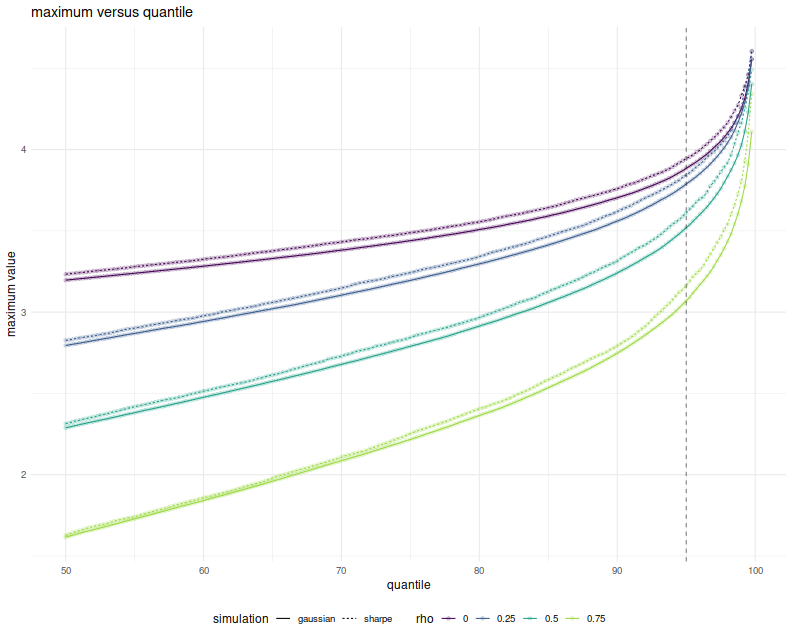The simulations here show what can happen when many strategies have mutual positive correlation. One might wonder what would happen if there were many strategies with a significant negative correlation. It turns out this is not really possible. In order for the correlation matrix to be positive definite, you cannot have too many strongly negative off-diagonal elements. Perhaps there is a Pigeonhole Principle argument for this, but a simple expectation argument suffices. Just in case, however, above I also simulated the case where you flip a coin to determine whether an element of the Gaussian has a positive or negative correlation to the common element. When the coin is completely biased to heads, you get the simulations shown above. When the coin is fair, the elements of the Gaussian are expected to be divided evenly into two highly groups. Here is the plot of the $$q$$th quantile of the maximum versus $$q$$, with different colors for $$\rho$$ and different lines for the probability of heads. Allowing negatively correlated elements does stochastically increase the maximum element, but never above the $$\rho=0$$ case. And so the Most Important Test still appears conservative. # plot max value vs quantile library(ggplot2) ph <- sumres %>% mutate(rho=factor(rho)) %>% mutate(prob. heads=factor(propcor)) %>% mutate(xv=as.numeric(gsub('%$','',qtile))) %>%
ggplot(aes(x=xv,y=value,color=rho,linetype=prob. heads)) +
geom_line() + geom_point(alpha=0.2) +
geom_vline(xintercept=95,linetype=2,alpha=0.5) +    # the 0.05 significance cutoff
labs(title='quantiles of maximum of B=1000 multivariate normal, various (anti) correlations',
x='quantile',y='maximum value')
print(ph)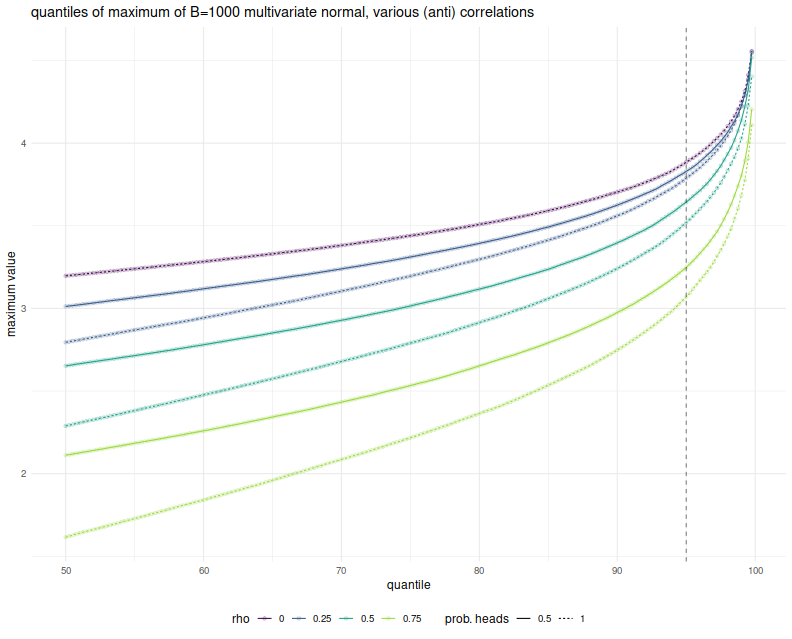In a followup post I will attempt to address the conservatism and hill-climbing issues, using the Markowitz approximation.

## The Sharpe Ratio is Biased

That the Sharpe ratio is biased is not unknown; this was established for Gaussian returns by Miller and Gehr in 1978. In the non-Gaussian case, the analyses of Jobson and Korkie (1981), Lo (2002), and Mertens (2002) have focused on the asymptotic distribution of the Sharpe ratio, via the Central Limit Theorem and the delta method. These techniques generally establish that the estimator is asymptotically unbiased, with some specified asymptotic variance.

The lack of asymptotic bias is not terribly comforting in our world of finite, sometimes small, sample sizes. Moreover, the finite sample bias of the Sharpe ratio might be geometric, which means that we could arrive at an estimator with smaller Mean Square Error (MSE), by dividing the Sharpe by some quantity. Finding the bias of the Sharpe seems like a straightforward application of Taylor's theorem. First we write

$$\hat{\sigma}^2 = \sigma^2 \left(1 + \frac{\hat{\sigma}^2 - \sigma^2}{\sigma^2}\right)$$

We can think of $$\epsilon = \frac{\hat{\sigma^2} - \sigma^2}{\sigma^2}$$ as the error, when we perform a Taylor expansion of $$x^{-1/2}$$ around $$1$$. That expansion is

$$\left(\sigma^2 \left(1 + \epsilon\right)\right)^{-1/2} \approx \sigma^{-1}\left(1 - \frac{1}{2}\epsilon + \frac{3}{8}\epsilon^2 + \ldots \right)$$

We can use linearity of expectation to get the expectation of the Sharpe ratio (which I denote $$\hat{\zeta}$$) as follows:

$$\operatorname{E}\left[\hat{\zeta}\right] = \zeta\left(1 - \frac{1}{2}\operatorname{E}\left[\hat{\mu} \frac{\hat{\sigma}^2 - \sigma^2}{\sigma^2} \right] + \frac{3}{8} \operatorname{E}\left[ \hat{\mu} \left(\frac{\hat{\sigma}^2 - \sigma^2}{\sigma^2} \right)^2 \right] + \ldots \right)$$

After some ugly computations, we arrive at

$$\operatorname{E}\left[\hat{\zeta}\right] \approx \left(1 + \frac{3}{4n} + \frac{3\kappa}{8n}\right) \zeta - \frac{1}{2n}s + \operatorname{o}\left(n^{-3/2}\right).$$

As the saying goes, a week of scratching out equations can save you an hour in the library (or 5 minutes on google). This computation has been performed before (and more importantly, vetted by an editor). The paper I should have read (and should have read a long time ago) is the 2009 paper by Yong Bao, Estimation Risk-Adjusted Sharpe Ratio and Fund Performance Ranking Under a General Return Distribution. Bao uses what he calls a 'Nagar-type expansion' (I am not familiar with the reference) by essentially splitting $$\hat{\mu}$$ into $$\mu + \left(\hat{\mu} - \mu\right)$$, then separating terms based on whether they contain $$\hat{\mu}$$ to arrive at an estimate with more terms than shown above, but greater accuracy, a $$\operatorname{o}\left(n^{-2}\right)$$ error.

(Bao goes on to consider a 'double Sharpe ratio', following Vinod and Morey. The double Sharpe ratio is a Studentized Sharpe ratio: the Sharpe minus it's bias, then divided by its standard error. This is the Wald statistic for the implicit hypothesis test that the Signal-Noise ratio (i.e. the population Sharpe ratio) is equal to zero. It is not clear why zero is chosen as the null value.)

Yong Bao was kind enough to share his Gauss code. I have translated it into R, hopefully without error. Below I run some simulations with returns drawn from the 'Asymmetric Power Distribution' (APD) with varying values of $$n$$, $$\zeta$$, $$\alpha$$ and $$\lambda$$. For each setting of the parameters, I perform $$10^5$$ simulations, then compute the bias of the sample Sharpe ratio, then I use Bao's formula and the simplified formula above, but plugging in sample estimates of the Sharpe, skew, kurtosis, and so on to arrive at feasible estimates. I then compute, over the 100K simulations, the mean empirical bias, and the mean feasible estimators. I then use the two formulae with the population values to get infeasible biases.

suppressMessages({
library(dplyr)
library(tidyr)
library(tibble)
# https://cran.r-project.org/web/packages/doFuture/vignettes/doFuture.html
library(doFuture)
registerDoFuture()
plan(multiprocess)
library(ggplot2)
})
# /* k-statistic, see Kendall's Advanced Theory of Statistics */
.kstat <- function(x) {
# note we can make this faster because we have subtracted off the mean
# so s=0 identically, but don't bother for now.
n <- length(x)
s <- unlist(lapply(1:6,function(iii) { sum(x^iii) }))
nn <- n^(1:6)
nd <- exp(lfactorial(n) - lfactorial(n-(1:6)))
k <- rep(0,6)
k <- s/n;
k <- (n*s-s^2)/nd;
k <- (nn*s-3*n*s*s+2*s^3)/nd
k <- ((nn+nn)*s-4*(nn+n)*s*s-3*(nn-n)*s^2 +12*n*s*s^2-6*s^4)/nd;
k <- ((nn+5*nn)*s-5*(nn+5*nn)*s*s-10*(nn-nn)*s*s
+20*(nn+2*n)*s*s^2+30*(nn-n)*s^2*s-60*n*s*s^3 +24*s^5)/nd;
k <- ((nn+16*nn+11*nn-4*nn)*s
-6*(nn+16*nn+11*nn-4*n)*s*s
-15*n*(n-1)^2*(n+4)*s*s-10*(nn-2*nn+5*nn-4*n)*s^2
+30*(nn+9*nn+2*n)*s*s^2+120*(nn-n)*s*s*s
+30*(nn-3*nn+2*n)*s^3-120*(nn+3*n)*s*s^3
-270*(nn-n)*s^2*s^2+360*n*s*s^4-120*s^6)/nd;

k
}
# sample gammas from observation;
# first value is mean, second is variance, then standardized 3 through 6
# moments
some_gams <- function(y) {
mu <- mean(y)
x <- y - mu
k <- .kstat(x)
retv <- c(mu,k,k[3:6] / (k ^ ((3:6)/2)))
retv
}
# /* Simulate Standadized APD */
.deltafn <- function(alpha,lambda) {
2*alpha^lambda*(1-alpha)^lambda/(alpha^lambda+(1-alpha)^lambda)
}
apdmoment <- function(alpha,lambda,r) {
delta <- .deltafn(alpha,lambda);
m <- gamma((1+r)/lambda)*((1-alpha)^(1+r)+(-1)^r*alpha^(1+r));
m <- m/gamma(1/lambda);
m <- m/(delta^(r/lambda));
m
}
# variates from the APD;
.rapd <- function(n,alpha,lambda,delta,m1,m2) {
W <- rgamma(n, scale=1, shape=1/lambda)
V <- (W/delta)^(1/lambda)
e <- runif(n)
S <- -1*(e<=alpha)+(e>alpha)
U <- -alpha*(V*(S<=0))+(1-alpha)*(V*(S>0))
Z <- (U-m1)/sqrt(m2-m1^2); # /* Standardized APD */
}
# to get APD distributions use this:
#rapd <- function(n,alpha,lambda) {
#   delta <- .deltafn(alpha,lambda)
#   # /* APD moments about zero */
#   m1 <- apdmoment(alpha=alpha,lambda=lambda,1);
#   m2 <- apdmoment(alpha=alpha,lambda=lambda,2);
#   Z <- .rapd(n,alpha=alpha,lambda=lambda,delta=delta,m1=m1,m2=m2)
#}

# /* From uncentered moment m to centered moment mu */
.m_to_mu <- function(m) {
n <- length(m)
mu <- m
for (iii in 2:n) {
for (jjj in 1:iii) {
if (jjj<iii) {
mu[iii] <- mu[iii] + choose(iii,jjj) * m[iii-jjj]*(-m)^jjj;
} else {
mu[iii] <- mu[iii] + choose(iii,jjj) * (-m)^jjj;
}
}
}
mu
}

# true centered moments of the APD distribution
.apd_centered <- function(alpha,lambda) {
m <- unlist(lapply(1:6,apdmoment,alpha=alpha,lambda=lambda))
mu <- .m_to_mu(m)
}
# true standardized moments of the APD distribution
.apd_standardized <- function(alpha,lambda) {
k <- .apd_centered(alpha,lambda)
retv <- c(1,1,k[3:6] / (k ^ ((3:6)/2)))
}
# true APD cumulants: skew, excess kurtosis, ...
.apd_r <- function(alpha,lambda) {
mustandardized <- .apd_standardized(alpha,lambda)
r <- rep(0,4)
r <- mustandardized
r <- mustandardized-3
r <- mustandardized-10*r
r <- mustandardized-15*r-10*r^2-15
r
}
# Bao's Bias function;
# use this in the feasible and infeasible.
.bias2 <- function(TT,S,r) {
retv <- 3*S/4/TT+49*S/32/TT^2-r*(1/2/TT+3/8/TT^2)+S*r*(3/8/TT-15/32/TT^2) +3*r/8/TT^2-5*S*r/16/TT^2-5*S*r^2/4/TT^2+105*S*r^2/128/TT^2-15*r*r/16/TT^2;
}
# one simulation.
onesim <- function(n,pzeta,alpha,lambda,delta,m1,m2,...) {
x <- pzeta + .rapd(n=n,alpha=alpha,lambda=lambda,delta=delta,m1=m1,m2=m2)
rhat <- some_gams(x)
Shat <- rhat / sqrt(rhat)
emp_bias <- Shat - pzeta
# feasible bias estimation
feas_bias_skew <- (3/(8*n)) * (2 + rhat) * Shat - (1/(2*n)) * rhat
feas_bias_bao <- .bias2(TT=n,S=Shat,r=rhat[3:6])
cbind(pzeta,emp_bias,feas_bias_skew,feas_bias_bao)
}
# many sims.
repsim <- function(nrep,n,pzeta,alpha,lambda,delta,m1,m2) {
jumble <- replicate(nrep,onesim(n=n,pzeta=pzeta,alpha=alpha,lambda=lambda,delta=delta,m1=m1,m2=m2))
retv <- aperm(jumble,c(1,3,2))
dim(retv) <- c(nrep * length(pzeta),dim(jumble))
colnames(retv) <- colnames(jumble)
invisible(as.data.frame(retv))
}
manysim <- function(nrep,n,pzeta,alpha,lambda,nnodes=7) {
delta <- .deltafn(alpha,lambda)
# /* APD moments about zero */
m1 <- apdmoment(alpha=alpha,lambda=lambda,1);
m2 <- apdmoment(alpha=alpha,lambda=lambda,2);

if (nrep > 2*nnodes) {
# do in parallel.
nper <- table(1 + ((0:(nrep-1) %% nnodes)))
retv <- foreach(i=1:nnodes,.export = c('n','pzeta','nu','alpha','lambda','delta','m1','m2',
'.kstat','some_gams','.rapd','onesim','repsim')) %dopar% {
repsim(nrep=nper[i],n=n,pzeta=pzeta,alpha=alpha,lambda=lambda,delta=delta,m1=m1,m2=m2)
} %>%
bind_rows()
} else {
retv <- repsim(nrep=nrep,n=n,pzeta=pzeta,alpha=alpha,lambda=lambda,delta=delta,m1=m1,m2=m2)
}
retv
}

ope <- 252
pzetasq <- c(0,1/4,1,4) / ope
pzeta <- sqrt(pzetasq)

apd_param <- tibble::tribble(~dgp,   ~alpha,  ~lambda,
'dgp7',    0.3,        1,
'dgp8',    0.3,        2,
'dgp9',    0.3,        5,
'dgp10',   0.7,        1,
'dgp11',   0.7,        2,
'dgp12',   0.7,        5)

params <- tidyr::crossing(tibble::tribble(~n,128,256,512),
tibble::tibble(pzeta=pzeta),
apd_param)

# run a bunch; on my machine, with 8 cores,
# 5000 takes ~68 seconds
#  1e4 takes ~2 minutes.
#  1e5 should take 20?
nrep <- 1e5
set.seed(1234)
system.time({
results <- params %>%
group_by(n,pzeta,dgp,alpha,lambda) %>%
summarize(sims=list(manysim(nrep=nrep,nnodes=8,
pzeta=pzeta,n=n,alpha=alpha,lambda=lambda))) %>%
ungroup() %>%
tidyr::unnest() %>%
dplyr::select(-pzeta1)
})

    user   system  elapsed
3818.083   40.473  549.383

# pop moment/cumulant function
pop_moments <- function(pzeta,alpha,lambda) {
rfoo <- .apd_r(alpha,lambda)
data_frame(mean=pzeta,var=1,skew=rfoo,exkurt=rfoo)
}
# Bao bias function
pop_bias_bao <- function(n,pzeta,alpha,lambda) {
rfoo <- .apd_r(alpha,lambda)
rhat <- c(pzeta,1,rfoo)
retv <- .bias2(TT=n,S=pzeta,r=rhat[3:6])
}
# attach population values and bias estimates
parres <- params %>%
group_by(n,pzeta,dgp,alpha,lambda) %>%
mutate(foo=list(pop_moments(pzeta,alpha,lambda))) %>%
ungroup() %>%
unnest() %>%
group_by(n,pzeta,dgp,alpha,lambda) %>%
mutate(real_bias_skew=(3/(8*n)) * (2 + exkurt) * pzeta - (1/(2*n)) * skew,
real_bias_bao =pop_bias_bao(n,pzeta,alpha,lambda)) %>%
ungroup()
# put them all together
sumres <- results %>%
group_by(dgp,pzeta,n,alpha,lambda) %>%
summarize(mean_emp=mean(emp_bias),
serr_emp=sd(emp_bias) / sqrt(n()),
mean_bao=mean(feas_bias_bao),
mean_thr=mean(feas_bias_skew)) %>%
ungroup() %>%
left_join(parres,by=c('dgp','n','pzeta','alpha','lambda'))
# done


Here I plot the empirical average biases versus the population skew and the population excess kurtosis. On the right facet we clearly see that Sharpe bias is decreasing in population skew. The choices of $$\alpha$$ and $$\lambda$$ here do not give symmetric and kurtotic distributions, so it seems worthwhile to re-test this with returns drawn from, say, the $$t$$ distribution. (The plot color corresponds to 'effect size', which is $$\sqrt{n}\zeta$$, a unitless quantity, but which gives little information in this plot.)

# plot empirical error vs cumulant
library(ggplot2)
ph <- sumres %>%
mutate(effect size=factor(signif(sqrt(n) * pzeta,digits=2))) %>%
rename(excess kurtosis=exkurt) %>%
tidyr::gather(key=moment,value=value,skew,excess kurtosis) %>%
mutate(n=factor(n)) %>%
ggplot(aes(x=value,y=mean_emp,color=effect size)) +
geom_jitter(alpha=0.3) +
geom_errorbar(aes(ymin=mean_emp - serr_emp,ymax=mean_emp + serr_emp)) +
facet_grid(. ~ moment,labeller=label_both,scales='free',space='free') +
labs(title='bias vs population cumulants',x='cumulant',y='empirical bias')
print(ph)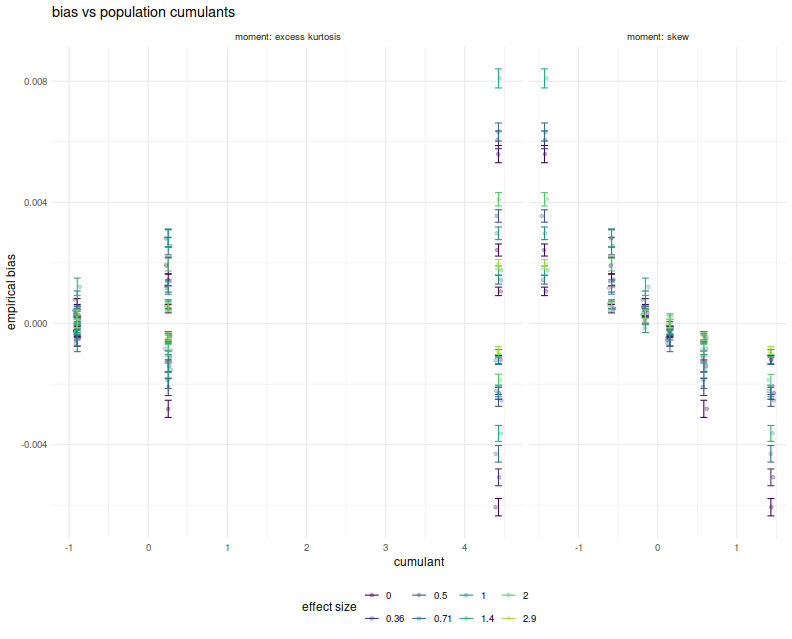Here I plot the mean feasible and infeasible bias estimates against the empirical biases, with different facets for $$\alpha, \lambda, n$$. Within each facet there should be four populations, corresponding to the Signal Noise ratio varying from 0 to $$4$$ annualized (yes, this is very high). I plot horizontal error bars at 1 standard error, and the $$y=x$$ line. There seems to be very little difference between the different estimators of bias, and they all seem to be very close to the $$y=x$$ line to consider them passable estimates of the bias.

# plot vs empirical error
ph <- sumres %>%
tidyr::gather(key=series,value=value,mean_bao,mean_thr,real_bias_skew,real_bias_bao) %>%
ggplot(aes(x=mean_emp,y=value,color=series)) +
geom_point() +
facet_grid(n + alpha ~ lambda,labeller=label_both,scales='free',space='free') +
geom_abline(intercept=0,slope=1,linetype=2,alpha=0.3) +
geom_errorbarh(aes(xmin=mean_emp-serr_emp,xmax=mean_emp+serr_emp,height=.0005)) +
theme(axis.text.x=element_text(angle=-45)) +
labs(title='bias',x='empirical bias',y='approximations')
print(ph)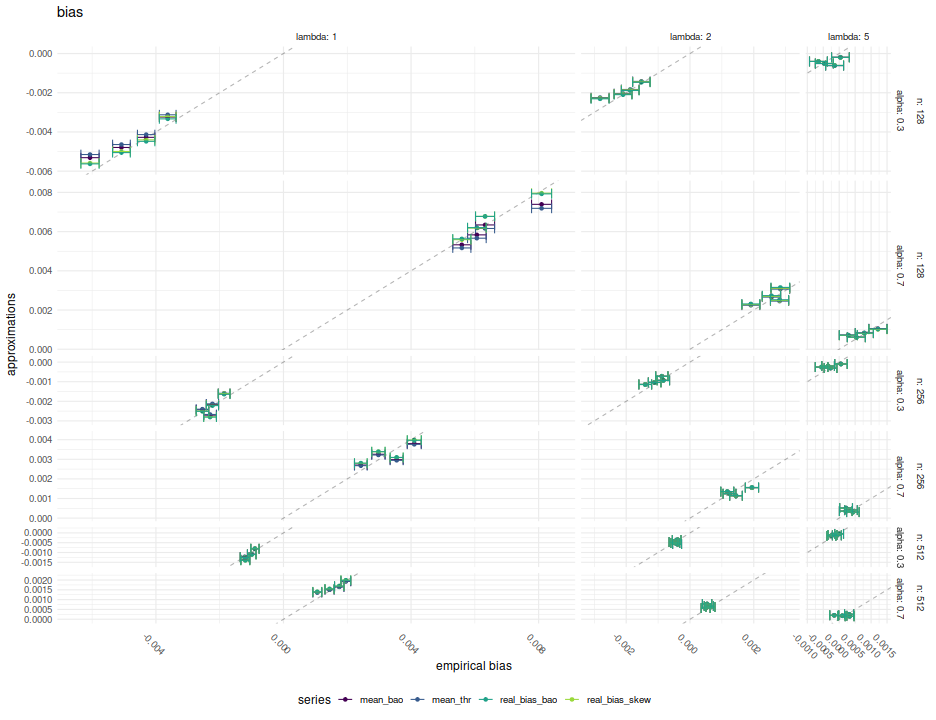Both the formula given above and Bao's formula seem to capture the bias of the Sharpe ratio in the simulations considered here. In their feasible forms, neither of them seems seriously affected by estimation error of the higher order cumulants, in expectation. I will recommend either of them, and hope to include them as options in SharpeR.

Note, however, that for the purpose of hypothesis tests on the Signal Noise ratio, say, that the bias is essentially $$\operatorname{o}\left(n^{-1}\right)$$ in the cumulants, but Mertens' correction to the standard error of the Sharpe is $$\operatorname{o}\left(n^{-1/2}\right)$$. That is, I expect very little to change in a hypothesis test by incorporating the bias term if Mertens' correction is already being used. Moreover, I expect using the bias term to have little improvement on the mean squared error, especially versus the drawdown estimator.

## A Sharper Sharpe: Just Shrink it!

In a series of blog posts we have looked at Damien Challet's 'Sharper estimator' of the Signal-Noise Ratio, finding it to have lower mean square error than the Sharpe ratio (which we are also calling the 'moment based estimator') for symmetric heavy tailed and skewed returns distributions; in a later post, we compared it to two other estimators for the case of $$t$$-distributed returns. In that last post we noticed that for a substantially easier problem (estimating the mean of a shifted, rescaled $$t$$ when the degrees of freedom and rescaling factor are known), the drawdown estimator seems to achieve lower mean square error than the Cramér Rao lower bound.

From this puzzling result, I suspected that the drawdown estimator is performing some kind of subconcious shrinkage. That is, the drawdown estimator estimates Signal Noise ratio by computing the average, under multiple permutations, number of drawdown and drawup records, then feeds these into a spline function which was built on simulations to map back to Signal Noise ratio. The problem is this reverse mapping may have been built on a limited parameter set with, say, a least squares fit. Values outside of the training range will be pulled back into the training range.

To be concrete, if the training simulations only included Signal Noise ratios between $$-1.5$$ and $$1.5$$ annualized, say, and the process were tested on returns with a Signal Noise ratio of $$3.5$$, say (these are comically large values for illustration purposes), then the spline function would very likely suggest the value is $$1.5$$. While this seems like a catastrope in our hypothetical situation, if you happen to test the estimator on populations which are within the range of Signal Noise ratios used in calibration, you are likely to see reduced mean square error, as we have in our simulations.

Rather than advocate for or against this particular choice of bias-variance tradeoff, here we consider an alternative shrinkage estimator: we take the vanilla Sharpe ratio and multiply it by 0.80. Here we test this basic shrinkage estimator against the Sharpe ratio and the drawdown estimator over a range of sample sizes, Signal Noise ratios, and kurtosis factor for returns drawn from normal or $$t$$ distributions, as in our first study. We compute the empirical root mean square error of each estimator along with the empirical bias over 500 simulations for each parameter setting.

suppressMessages({
library(dplyr)
library(tidyr)
library(tibble)
library(sharpeRratio)
# https://cran.r-project.org/web/packages/doFuture/vignettes/doFuture.html
library(doFuture)
registerDoFuture()
plan(multiprocess)
})

onesim <- function(n,pzeta,gen=rnorm,...) {
x <- gen(n) + pzeta
mux <- mean(x)
sdx <- sd(x)
mome <- (mux + (pzeta - pzeta)) / sdx
sim$SNR })) cbind(pzeta,mome,ddsr) } repsim <- function(nrep,n,pzeta,gen=rnorm) { dummy <- invisible(capture.output(jumble <- replicate(nrep,onesim(n=n,pzeta=pzeta,gen=gen)),file='/dev/null')) retv <- aperm(jumble,c(1,3,2)) dim(retv) <- c(nrep * length(pzeta),dim(jumble)) colnames(retv) <- colnames(jumble) invisible(as.data.frame(retv)) } manysim <- function(nrep,n,pzeta,ex.kurt=0,nnodes=5) { stopifnot(ex.kurt >= 0) # not yet if (ex.kurt==0) { gen <- rnorm } else { thedf <- 4 + 6 / ex.kurt rescal <- sqrt((thedf - 2)/thedf) gen <- function(n) { rescal * rt(n,df=thedf) } } if (nrep > 2*nnodes) { # do in parallel. nper <- table(1 + ((0:(nrep-1) %% nnodes))) retv <- foreach(i=1:nnodes,.export = c('n','pzeta','gen','onesim','repsim')) %dopar% { repsim(nrep=nper[i],n=n,pzeta=pzeta,gen=gen) } %>% bind_rows() } else { retv <- repsim(nrep=nrep,n=n,pzeta=pzeta,gen=gen) } retv } ope <- 252 pzetasq <- c(0,1/4,1,4) / ope pzeta <- sqrt(pzetasq) params <- tidyr::crossing(tibble::tribble(~n,100,200,400,800,1600), tibble::tribble(~kurty,0,4,16,64), tibble::tibble(pzeta=pzeta)) # run it nrep <- 500 set.seed(1234) system.time({ results <- params %>% group_by(n,kurty,pzeta) %>% summarize(sims=list(manysim(nrep=nrep,nnodes=6, pzeta=pzeta,n=n,ex.kurt=kurty))) %>% ungroup() %>% tidyr::unnest() })   user system elapsed 12503.75 4537.97 2532.52  Now I collect the results of the simulation, computing the bias, standard error and root mean square error of the two methods of computing Sharpe. # summarize the moments shrinkage_amt <- 0.80 sumres <- results %>% mutate(shrn=shrinkage_amt * mome) %>% # "shrinkage" group_by(pzeta,n,kurty) %>% summarize(bias_mome=mean(mome - pzeta,na.rm=TRUE), rmse_mome=sqrt(mean((mome - pzeta)^2,na.rm=TRUE)), bias_shrn=mean(shrn - pzeta,na.rm=TRUE), rmse_shrn=sqrt(mean((shrn - pzeta)^2,na.rm=TRUE)), bias_ddsr=mean(ddsr - pzeta,na.rm=TRUE), rmse_ddsr=sqrt(mean((ddsr - pzeta)^2,na.rm=TRUE))) %>% ungroup() %>% arrange(pzeta,n,kurty) %>% tidyr::gather(key=series,value=value,matches('_(mome|ddsr|shrn)$')) %>%
tidyr::separate(series,into=c('metric','stat')) %>%
mutate(stat=case_when(.$stat=='mome' ~ 'moment based estimator', .$stat=='ddsr' ~ 'drawdown based estimator',
.\$stat=='shrn' ~ 'shrunk moment based estimator',
mutate(annualized SNR=signif(pzeta * sqrt(ope),digits=2)) %>%
rename(excess kurtosis=kurty)


Here we plot the RMSE versus sample size. The new shrinkage moment based estimator, plotted in blue, appears to achieve about the same mean square error than the drawdown based estimator, sometimes a little lower, sometimes a little higher.

# plot
library(ggplot2)
ph <- sumres %>%
filter(metric=='rmse') %>%
mutate(value=sqrt(ope) * value) %>% # annualized
ggplot(aes(n,value,color=stat)) +
geom_line() + geom_point() +
scale_x_log10() +
scale_y_log10() +
facet_grid(annualized SNR~excess kurtosis,labeller=label_both) +
labs(y='RMSE of estimator of SNR, annualized',
x='number of days of data',
title='empirical root mean squared errors of three ways of estimating Signal Noise ratio')
print(ph)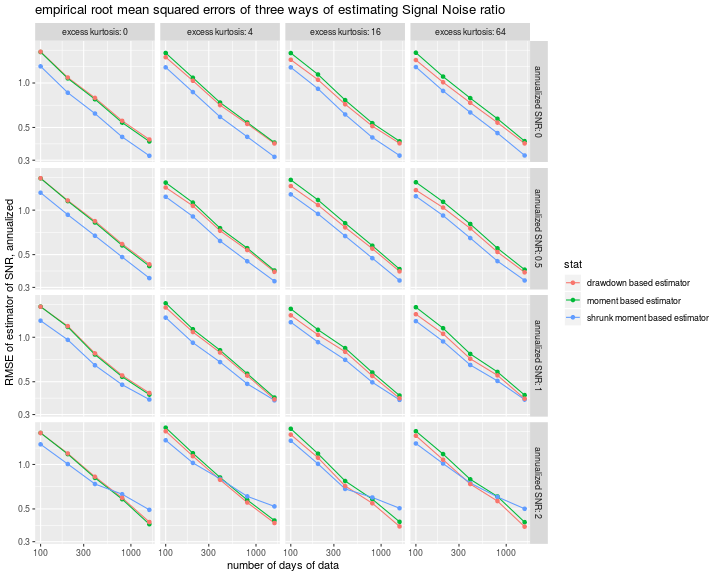Here we plot the bias (in annualized units) of the three estimators. The traditional Sharpe ratio is the only estimator among the three that appears to be nearly unbiased. The drawdown estimator is nearly unbiased for the case of normal returns, but has about the same sizable bias for $$t$$ returns.

# plot
library(ggplot2)
ph <- sumres %>%
filter(metric=='bias') %>%
mutate(value=sqrt(ope) * value) %>% # annualized
ggplot(aes(n,value,color=stat)) +
geom_line() + geom_point() +
scale_x_log10() +
facet_grid(annualized SNR~excess kurtosis,labeller=label_both) +
labs(y='bias of estimator of SNR, annualized',
x='number of days of data',
title='empirical bias of three ways of estimating Signal Noise ratio')
print(ph)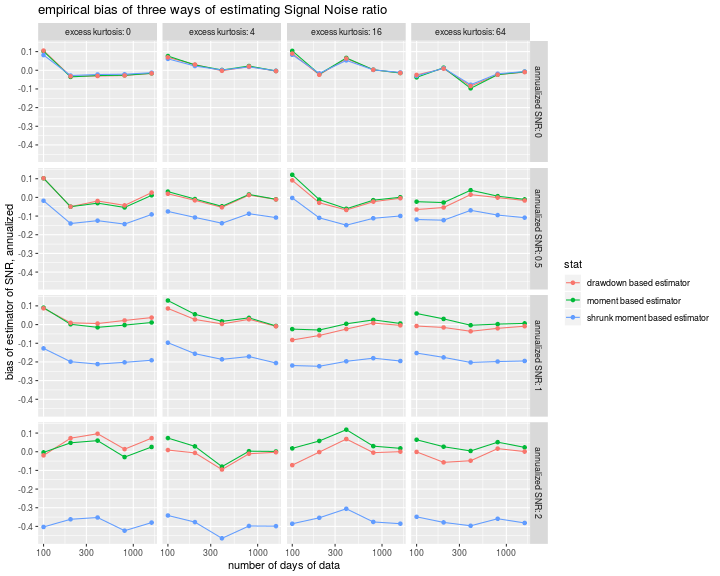We are not suggesting that quant managers take a 20% haircut off of their Sharpe ratios! (Although perhaps that's reasonable for backtests.) The point is that a very simple, and truly repugnant, modification to the Sharpe ratio can achieve the same empirical performance as the drawdown estimator. At the same time, the latter lacks any theoretical justification for improved efficiency. Together these cast serious doubts on the drawdown estimator for practical use.